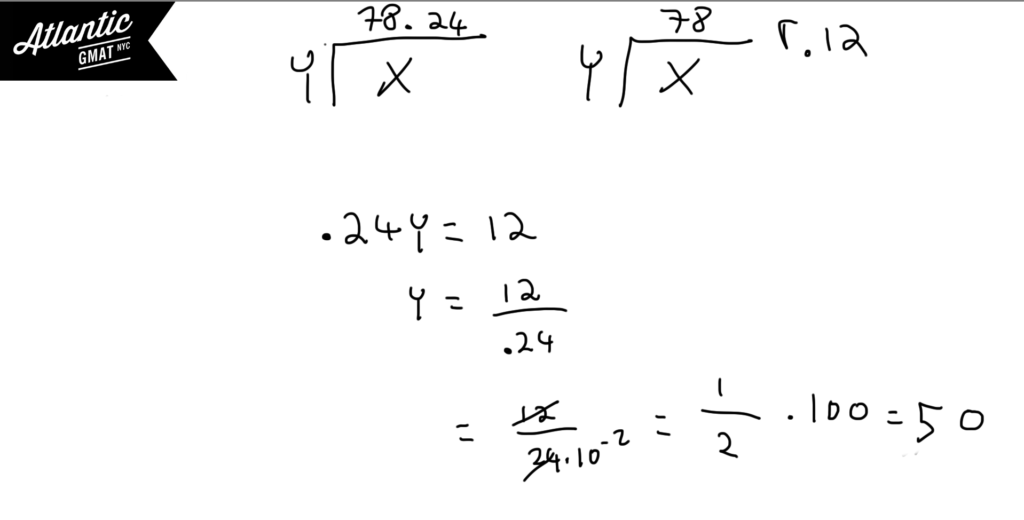Private GMAT Tutoring in NYC & Online

# GMAT Question of the Day - Problem Solving - Remainder

x and y are positive integers. When x is divided by y the remainder is 12. x/y = 78.24. What is the value of y?

A. 50

B. 52

C. 58

D. 62

E. 66

## GMAT Question of the Day Solution

If you know the remainder formula this question will be simple. The basic idea is that y multiplied by the decimal part of the quotient (.24) must equal the remainder (12). It will be helpful to memorize this relationship. In your arithmetic you can use powers of 10 to simplify the decimal or just convert the decimal to a fraction.# CONTACT

Atlantic GMAT

405 East 51st St.

NY, NY 10022

(347) 669-3545

info@AtlanticGMAT.com